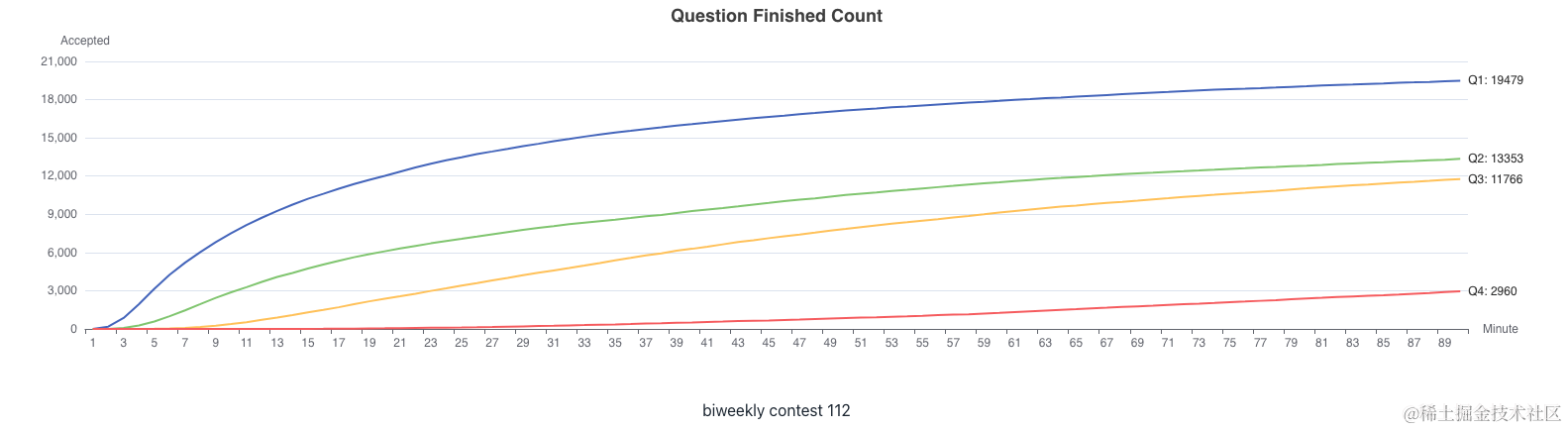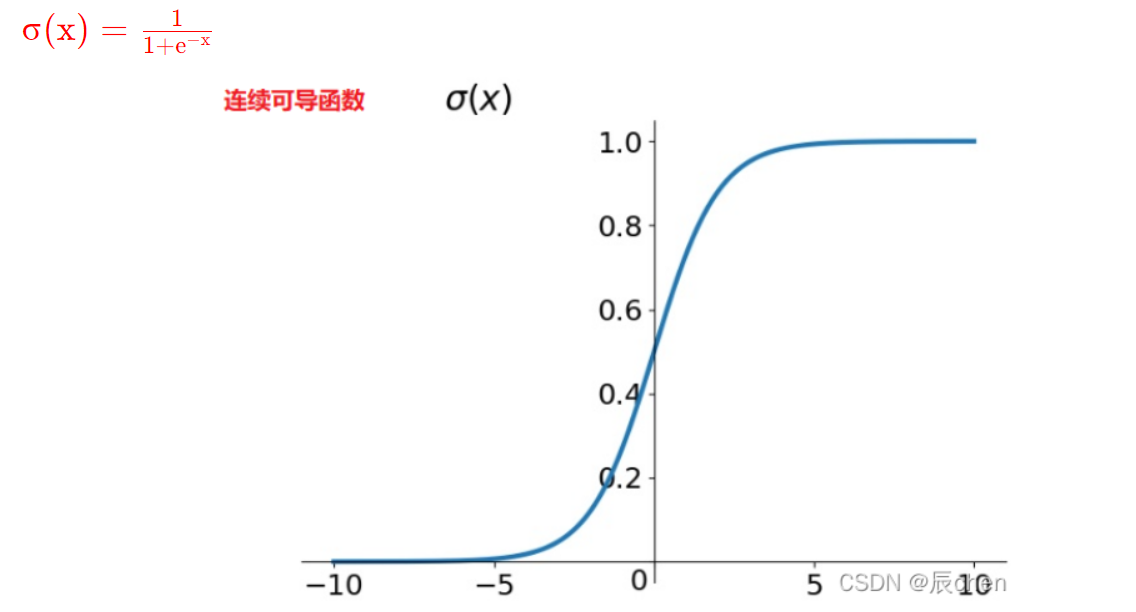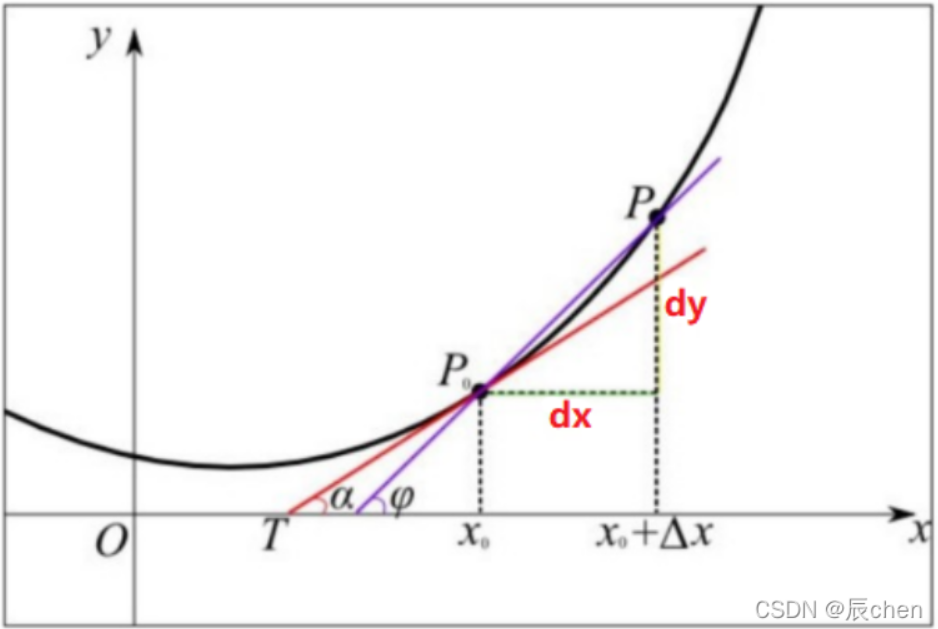# 每个程序员都应该知道的基础数论

+关注继续查看0. 皮亚诺公理

• 0是自然数;
• 每个自然数都有一个后续自然数;
• 0不是任何自然数的后续自然数;
• 不同自然数的后续自然数不同;
• 如果集合S包含了数字0，并且包含S中每一个数字的后续自然数，那么集合S就包含了所有的自然数。

1. 算术基本定理和除法运算法则

• a = bq + r， 0 <= r < b

2. 欧几里得定理

• 第一定理：p|ab => p|a or p|b。该定理的直接结论就是算术基本定理。
• 第二定理：质数的数量是无限的。有很多简单的证明方法。

3. 最大公约数、最小公倍数和贝祖定理

• 如果 (a,b) = 1，即 [a,b] = ab，此时我们称a和b互质。
• 如果 (a,b) = d，那么 (a/d,b/d) = 1。

4. 整数因式分解

5. 线性同余方程组

• da'x + dn'( - y)= dd'，其中gcd(a'，n')= 1
• 带入变量d
• a'x + n'( - y)= d'。
• 由于gcd(a'，n') = 1，现在我们可以使用扩展的欧几里德的算法来找到a'x + n'( - y)= 1的解，然后将该解乘以d'得到对于a'x + n'( - y)= d'的解。

6.中国剩余定理

• x ≡ a1 (mod n1)
• x ≡ a2 (mod n2)
• x ≡ a3 (mod n3)
• ....
• x ≡ ak (mod nk)

• c = a1c1d1 + a2c2d2 + ... + akckdk

• x≡a1(mod n1)
• x≡a2(mod n2)
• x≡a3(mod n3)
• ....
• x≡ak(mod nk)

7. 二次方一致性

8. 欧拉 Phi 函数、除数函数、约数和、Mobius 函数

•  如果 p 是素数，则 φ(pk) = (p-1)pk-1
• φ 函数是乘法的，即如果 if (a,b) = 1 则 φ(ab) = φ(a)φ(b)。
•  φ(n) 的值可以通过欧拉公式获得：令 n = p1a1 * p2a2 * .... * pkak 是 n 的素因子分解。则

1. φ(n) = n * (1- 1/p1)) * (1- 1/p2)) * ... * (1- 1/pk))
• 以编程方式，如果我们欲求 1 到 n 的 φ ， 那么我们可以非常好地使用筛选算法连同 φ 的乘法性质。中心思想是：如果 n 是素数，则 φ(n) = n-1。否则，如果 n 是素数的幂，例如 n= pk，则 φ(n) = (p-1)pk-1。否则，对于某个素数p，令 n=pk*q 。使用乘法属性， 我们有 φ(n) = φ(pk)φ(q)

φ(n) 的两个重要属性：

• i. aφ(n) ≡ 1 (mod n) 每当 (a,n) = 1。 具体来说， 对于素数p，如果 p 不能整除 a，则 ap-1 ≡ 1 (mod p)。 这种特化也被称为费马小定理。
• ii. 令 d1, d2, ...dk 为 n 的所有除数(包括 n)。则 φ(d1) + φ(d2) + ... + φ(dk) = n

• 如果 p 是素数，则 d(p) = 2。另外， d(pk) = k+1， 并且 σ(p) = p+1
• 如果 n 是两个不同的素数的乘积，假使 n = pq， 则 σ(n) = n+1+(p+q)。另外观察到这种情况：φ(n) = n+1-(p+q)。
• 一般来说，令 n = p1a1 * p2a2 * .... * pkak 。则 d(n) = (a1+1) * (a2+1) * ... (ak + 1)，并且 σ(n) 由以下乘积给出：

1. σ(n) = ( (p1(a1+1) - 1) / (p1-1) ) * ( (p2(a2+1) - 1) / (p2-1) ) * ... * ( (pk(ak+1) - 1) / (pk-1) )

mobius函数µ(n) 在所有正整数中定义如下：

• 在 n 是非平方数(即 n 是不能被任意整数平方得到)并且 n 有偶数个不同的素数因子，则 µ(n) = 1
• 在 n 是非平方数(即 n 是不能被任意整数平方得到)并且 n 具有奇数个不同素数因子，则µ(n) = -1
• 在 n 是平方数，即 n 是某个整数的平方，则 µ(n) = 0

Mobius 函数是乘法分配性的，即 a 和 b 互为质数，则 µ(ab) = µ(a)*µ(b).

• φ(n) = (d1 * mu (n/d1) ) + (d2 * µ(n/d2) ) + .... + (dk * µ(n/dk) )

• φ(n) = (µ(d1) * (n/d1) ) + (µ(d2) * (n/d2) ) + .... + (µ(dk) * (n/dk) )

2. int phi[] = new int[n+1];
3. for(int i=2; i <= n; i++) phi[i] = i; //phi is 0
4. for(int i=2; i <= n; i++)
5. if( phi[i] == i )
6. for(int j=i; j <= n; j += i )
7. phi[j] = (phi[j]/i)*(i-1);

• 对数论的介绍：哈迪和赖特
• 初等数论: 琼斯和琼斯
• 数学诱导 - 一种技术教程，经常用于离散空间的证明。|
29天前
|

LeetCode 周赛上分之旅 #43 计算机科学本质上是数学吗？

20 0|
2月前
|

41 1
|
10月前
|

111 0
|

134 0|

154 0|

76 0|

一些著名问题与算法 　　如果您的飞船破了一个洞，我只能深表同情，因为我所解决的99个问题里唯独没有这个问题。 　　——匿名者1 　　本文提到的所有问题与算法，因为有一些算法仅仅是为了试图说明某个原理，而有一些问题仅仅是为了某个算法而创造的。然而，作为索引，这里会列举出学习中最重要的那些问题与算法。 　　在本文大多数描述中，n代表的是问题规模，如一个序列中的元素数量。而在图论问题中，n表示的是节点的数量，m则表示边的数量。
157 0
|

63 0